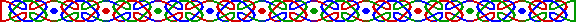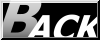an-1 = 1 (mod n) 而對於所有 i ， a(n-1)/qi 不等於 1 (mod n)。則 n 為素數。

24092/11=3024 (mod 4093)，24092/31=1121 (mod 4093)，因此 4093 是素數。2n-1 = 1 (mod n)，21000 不等於 1 (mod 823001)，數值答案從略。從普羅絲定理可知 823001 是素數。ain-1 = 1 (mod n)及 (ai(n-1)/qi-1 , n) = 1，則 n 的每一個素因子 p 都滿足 p=1 (mod F)。n < (mF1 + 1) [2F12 + (r-m)F1 + 1]

R1= 2 F1 s + r，r 介乎 1 至 2 F1 之間

(mF1 + 1) [2F12 + (r-m)F1 + 1] = 3333331 * (2*33333302 + 3666666*3333330 + 1)

= 3333331 * (103333326 * 3333330+1) = 3333331 * 34444385555581 > n。1. 從 n-1、n+1或其他含 n之多項式 入手，因其是偶合數，要分解的話比分解奇數易得多。

2. 我們不須把目標數字完全分解，也能判斷其素性。Hosted by www.Geocities.ws